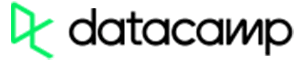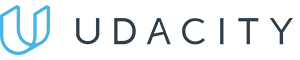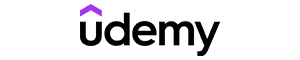Code has been added to clipboard!

# JavaScript parseInt() Explained With Examples

Published Aug 10, 2017
Updated Oct 10, 2019

We have already covered that there are a bunch of different data types in JavaScript. One of the simplest data types is a number. It contains numerical data. Unlike in PHP, the same data type is used for both numbers with and without decimal points.

The JavaScript parseint function is handy when you need to quickly convert a string to numbers. In this tutorial, you will be introduced to the proper syntax of this method and get a few code examples to study. We will also be covering the radix parameter, which defines the numeral system your function will use.

## JavaScript parseInt: Main Tips

• JS `parseInt()` function makes JavaScript convert string to integer. A radix parameter is used to define the numeral system that is used. These are represented by a number from 2 to 36.
• If the radix parameter is omitted, JavaScript assumes it is 16 (hexadecimal) if a strings starts with `0x`, 8 (octal) if the string starts with `0` (deprecated), or 10 (decimal) if the string starts with any other value.
• Only the first string number is returned (trailing and leading spaces are allowed).
• In case the first symbol cannot be changed to a number in any way, `parseInt()` JavaScript returns `NaN`.Pros
• Easy to use with a learn-by-doing approach
• Offers quality content
• Gamified in-browser coding experience
• The price matches the quality
• Suitable for learners ranging from beginner to advanced
Main Features
• Free certificates of completion
• Focused on data science skills
• Flexible learning timetablePros
• Simplistic design (no unnecessary information)
• High-quality courses (even the free ones)
• Variety of features
Main Features
• Nanodegree programs
• Suitable for enterprises
• Paid Certificates of completionPros
• Easy to navigate
• No technical issues
• Seems to care about its users
Main Features
• Huge variety of courses
• 30-day refund policy
• Free certificates of completion

## Syntax and Code Examples

The syntax of JavaScript `parseInt()` is very simple.

• define the JS parseInt function: `parseInt`.
• open the parenthesis `(`.
• define a string, put a comma, define the radix: `string, radix`.
• close the parenthesis `)`.

The final result of JavaScript parse int syntax should look like this:

`parseInt(string, radix)`

To understand what JS `parseInt()` is, it is essential to see the examples. Take a look at this snippet of code in which we make JavaScript convert string to integer a few times:

Example
``````function learnFunction() {
var a = parseInt("20") + "<br>";
var b = parseInt("20.00") + "<br>";
var c = parseInt("20.66") + "<br>";
var d = parseInt("43 54 77") + "<br>";
var e = parseInt("   70   ") + "<br>";
var f = parseInt("30 years") + "<br>";
var g = parseInt("He is 30") + "<br>";

var x = a + b + c + d + e + f + g;
document.getElementById("learn").innerHTML = x;
}``````

Below is another example of string parsing with JavaScript `parseInt()` function:

Example
``````function learnFunction() {
var a = parseInt("20", 20) + "<br>";
var b = parseInt("010") + "<br>";
var c = parseInt("20", 7) + "<br>";
var d = parseInt("0x10") + "<br>";
var e = parseInt("10", 17) + "<br>";

var x = a + b + c + d + e;
document.getElementById("learn").innerHTML = x;
}``````

#### Parameter Values

parseint JavaScript function can have two parameters: the string you want to convert, and the radix which identifies the numeral system.

Parameter Description
string Needed. The string you want to be parsed
radix Not required. The numeral system represented by a number from 2 to 36

## JavaScript parseInt: Summary

• JavaScript parse int function is used to return the integer by parsing a string.
• Using `parseInt` JavaScript only returns the first number of the string.
• As a follow-up to this quick tutorial, you should check JavaScript Number Methods Tutorial.

Days
Hours
Minutes
Seconds